# [F/OS] 🖼️ ImageUtil - Additional Tools To The Image Component!Introduction

A non-visible extension that provides additional tools to the built-in Image component.Package name: com.gordonlu.imageutil.aixVersion: 5Release date: 2022-03-08T06:00:00ZMinimum API: 19 (Android 4.4 KitKat)Documentation

Method blocks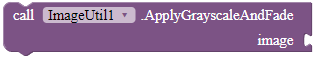Applies grayscale effect to an image and fades the image.

Parameters: component = image

ApplyWatermark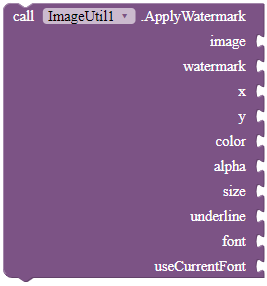Applies watermark text to the given image's content. Set the text of the watermark via the watermark parameter, and the x and y parameters are the co-ordinates of the watermark located on the image. The parameter color is the text color of the watermark, and alpha is the luminance of the watermark. The size parameter is the size of the watermark in points. Specify whether to underline the watermark via the underline boolean parameter. Use the blocks in the properties of this extension for the font parameter. If useCurrentFont is true, the font parameter will be ignored.

Parameters: image = component, watermark = text, x = number (int), y = number (int), color = color, alpha = number (int), size = number (int), underline = boolean, font = text, useCurrentFont = boolean

ColorBoost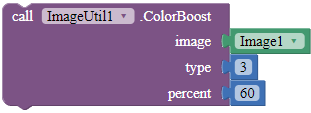Applies color boost to the content of the given content. To find out the type parameter, go to: Color Boost the image(Bitmap) in ImageView (Android) ~ Hamad's blog | Android News,Tutorials and Code-snippets. type should be a value, either 1, or 2, or 3.

Parameters: image = component, type = number (int), percent = number (float)

GammaEffect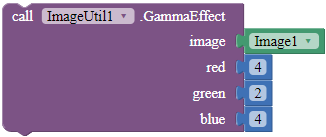Applies gamma effect to the given image.

Parameters: image = component, red = number (double), green = number (double), blue = number (double)

HueFilter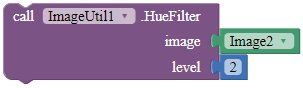Sets the hue filter for the image, with the given level.

Parameters: image = component, level = number (int)

ImageAlpha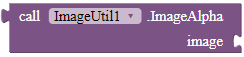Returns the alpha that was applied to this ImageView. This will probably be a value between 0 and 255.

Returns: number (int)

Parameters: image = component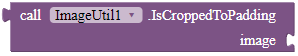Checks whether this image is cropped to padding.

Returns: boolean

Parameters: image = component

RoundCorners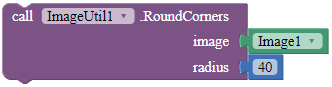Applies round corners to the Image, with the radius parameter as the radius of each corner.

Parameters: image = component, radius = number (int)

SepiaToningEffect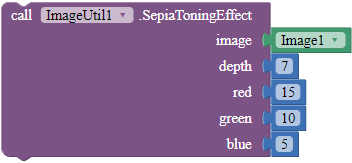Turns the image to a Sephia Toned version of the image, by specifying the depth, red, green and blue parameters.

Parameters: image = component, depth = number (int), red = number (double), green = number (double), blue = number (double)

SetBrightness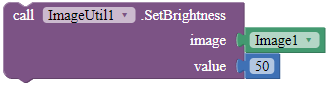Sets the brightness of the content for the given image, according to the value parameter.

Parameters: image = component, value = number (int)

SetColorDepth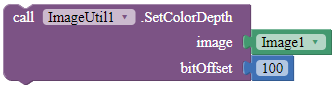Sets the color depth of the image.

Parameters: image = component, bitOffset = number (int)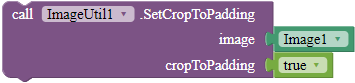Sets whether the image should crop to padding.

Parameters: image = component, cropToPadding = boolean

SetImageAlpha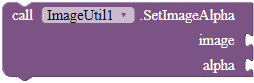Sets the alpha value that should be applied to the image.

Parameters: image = component, alpha = number (int)

SetImageTintColor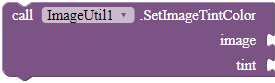Change the image tint color. You can use too alpha values if you want with the 'make a list' block. Do not forget to use the 'make color' block together with the 'make a list' block.

Parameters: image = component, tint = color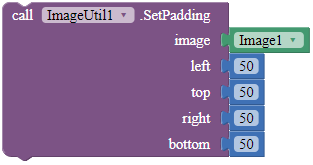Sets the padding of the given image.

Parameters: image = component, left = number (int), top = number (int), right = number (int), bottom = number (int)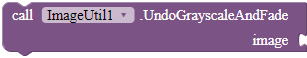Parameters: image = componentDownloads

AIX:
com.gordonlu.imageutil.aix (14.8 KB)

For the source code, here you go. Go to the GitHub repo, and don't forget toit!Rate my extension!• Good extension!

0 voters

KindlyPM me if you have any questions! Also, if you like my extension, pleaselike it! It takes some effort for me to make it...

Votes and likes tell me the general user feedback of my extension. If you read this extension, please take 20 seconds to drop by and give a vote / like!

If you have any features that you want to add and you know the code, PM me or directly reply below using the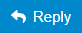button.

Gordon Lu

8 Likes

tests:

Companion

aiStarter emulator - does not work.

Xiaomi RedMi Note 10 - Works.

Profile.aia (87.4 KB)

interestingly, during testing, i figured out how to turn the image to black-and-white.

and then undo it via the UndoGrayscaleAndFade method.

1 Like

Two new methods added in version 2.

ApplyWatermark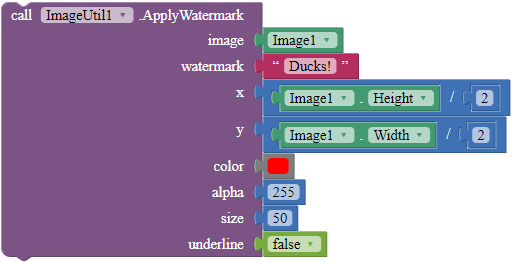Applies watermark text to the given image's content.

Parameters: image = component, watermark = text, x = number (int), y = number (int), color = color, alpha = number (int), size = number (int), underline = boolean

RoundCornersApplies round corners to the Image, with the radius parameter as the radius of each corner.

Parameters: image = component, radius = number (int)

1 Like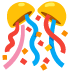Version 3!

• Updated ApplyWatermark. You can now set the watermark font to Serif, Monospace, Default and Sans Serif!

ApplyWatermarkApplies watermark text to the given image's content. Set the text of the watermark via the watermark parameter, and the x and y parameters are the co-ordinates of the watermark located on the image. The parameter color is the text color of the watermark, and alpha is the luminance of the watermark. The size parameter is the size of the watermark in points. Specify whether to underline the watermark via the underline boolean parameter. Use the blocks in the properties of this extension for the font parameter. If useCurrentFont is true, the font parameter will be ignored.

Parameters: Parameters: image = component, watermark = text, x = number (int), y = number (int), color = color, alpha = number (int), size = number (int), underline = boolean, font = text, useCurrentFont = boolean

By defining the font parameter, say hello to these properties.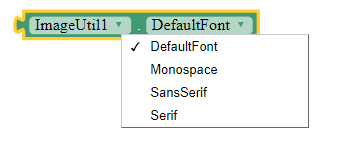• Cool effects for your images!

ColorBoostApplies color boost to the content of the given content. To find out the type parameter, go to: Color Boost the image(Bitmap) in ImageView (Android) ~ Hamad's blog | Android News,Tutorials and Code-snippets. type should be a value, either 1, or 2, or 3.

Parameters: image = component, type = number (int), percent = number (float)

GammaEffectApplies gamma effect to the given image.

Parameters: image = component, red = number (double), green = number (double), blue = number (double)Version 4!

• Some more cool effects for your Images!

SepiaToningEffectTurns the image to a Sephia Toned version of the image, by specifying the depth, red, green and blue parameters.

Parameters: image = component, depth = number (int), red = number (double), green = number (double), blue = number (double)

SetBrightnessSets the brightness of the content for the given image, according to the value parameter.

Parameters: image = component, value = number (int)Sets whether the image should crop to padding.

Parameters: image = component, cropToPadding = booleanSets the padding of the given image.

Parameters: image = component, left = number (int), top = number (int), right = number (int), bottom = number (int)

EDIT 1: This extension is now added on GitHub.Version 5!

• More cool effects for your images!

HueFilterSets the hue filter for the image, with the given level.

Parameters: image = component, level = number (int)

SetColorDepthSets the color depth of the image.

Parameters: image = component, bitOffset = number (int)

2 Likes